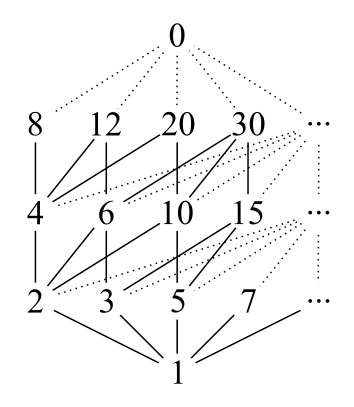"Double" DivisorsIf a three-digit number $\overline{abc}$ has 28 positive divisors, how many positive divisors does the six-digit number $\overline{abcabc}$ have?

×